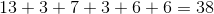ISEE Lower Level Quantitative : Plane Geometry

Example Questions

Example Question #77 : Geometry

What is the perimeter of the polygon below?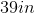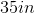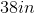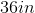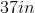Explanation:

To find the area of a perimeter, we add all of the side lengths together.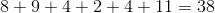Example Question #78 : Geometry

What is the perimeter of the polygon below?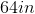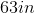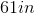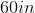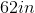Explanation:

To find the area of a perimeter, we add all of the side lengths together.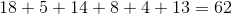Example Question #79 : Geometry

What is the perimeter of the polygon below?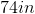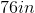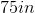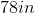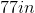Explanation:

To find the area of a perimeter, we add all of the side lengths together.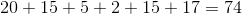Example Question #71 : Geometry

What is the perimeter of the polygon below?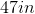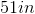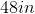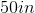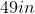Explanation:

To find the area of a perimeter, we add all of the side lengths together.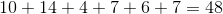Example Question #71 : Geometric Measurement: Recognizing Perimeter

What is the perimeter of the polygon below?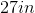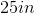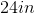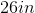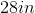Explanation:

To find the area of a perimeter, we add all of the side lengths together.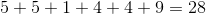Example Question #31 : How To Find The Perimeter Of A Figure

What is the perimeter of the polygon below?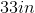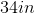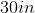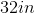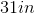Explanation:

To find the area of a perimeter, we add all of the side lengths together.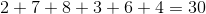Example Question #83 : Geometry

What is the perimeter of the polygon below?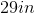Explanation:

To find the area of a perimeter, we add all of the side lengths together.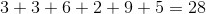Example Question #81 : Find Perimeter Or Missing Side Lengths Of Polygons: Ccss.Math.Content.3.Md.D.8

What is the perimeter of the polygon below?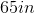Explanation:

To find the area of a perimeter, we add all of the side lengths together.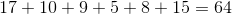Example Question #82 : Find Perimeter Or Missing Side Lengths Of Polygons: Ccss.Math.Content.3.Md.D.8

What is the perimeter of the polygon below?Explanation:

To find the area of a perimeter, we add all of the side lengths together.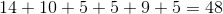Example Question #83 : Find Perimeter Or Missing Side Lengths Of Polygons: Ccss.Math.Content.3.Md.D.8

What is the perimeter of the polygon below?